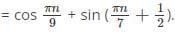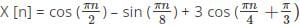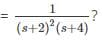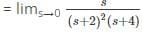Test: Discrete-Time Fourier Transform

# Test: Discrete-Time Fourier Transform

Test Description

## 10 Questions MCQ Test Signals and Systems | Test: Discrete-Time Fourier Transform

Test: Discrete-Time Fourier Transform for Electronics and Communication Engineering (ECE) 2023 is part of Signals and Systems preparation. The Test: Discrete-Time Fourier Transform questions and answers have been prepared according to the Electronics and Communication Engineering (ECE) exam syllabus.The Test: Discrete-Time Fourier Transform MCQs are made for Electronics and Communication Engineering (ECE) 2023 Exam. Find important definitions, questions, notes, meanings, examples, exercises, MCQs and online tests for Test: Discrete-Time Fourier Transform below.
Solutions of Test: Discrete-Time Fourier Transform questions in English are available as part of our Signals and Systems for Electronics and Communication Engineering (ECE) & Test: Discrete-Time Fourier Transform solutions in Hindi for Signals and Systems course. Download more important topics, notes, lectures and mock test series for Electronics and Communication Engineering (ECE) Exam by signing up for free. Attempt Test: Discrete-Time Fourier Transform | 10 questions in 30 minutes | Mock test for Electronics and Communication Engineering (ECE) preparation | Free important questions MCQ to study Signals and Systems for Electronics and Communication Engineering (ECE) Exam | Download free PDF with solutions
 1 Crore+ students have signed up on EduRev. Have you?
Test: Discrete-Time Fourier Transform - Question 1

### Given a discrete time signal x[k] defined by x[k] = 1, for -2 ≤ k ≤ 2 and 0, for |k| > 2. Then, y[k] = x[3k - 2] is ______________

Detailed Solution for Test: Discrete-Time Fourier Transform - Question 1

y[k] = x [3k-2]
Now, y  = x [-2] = 1
Or, y  = x  = 1
Or, y  = x  = 0
∴ y[k] = 1, for k = 0, 1 and 0 otherwise.

Test: Discrete-Time Fourier Transform - Question 2

### A discrete time signal is given as X [n]The period of the signal X [n] is ______________

Detailed Solution for Test: Discrete-Time Fourier Transform - Question 2

Given that, N1 = 18, N2 = 14
We know that period of X [n] (say N) = LCM (N1, N2)
∴ Period of X [n] = LCM (18, 14) = 126.

Test: Discrete-Time Fourier Transform - Question 3

### F(t) and G(t) are the one-sided z-transforms of discrete time functions f(nt) and g(nt), the z-transform of ∑f(kt)g(nt-kt) is given by _____________

Detailed Solution for Test: Discrete-Time Fourier Transform - Question 3

Given that F (t) and G (t) are the one-sided z-transforms.
Also, f (nt) and g (nt) are discrete time functions, which means that property of Linearity, time shifting and time scaling will be similar to that of continuous Fourier transform. Since, for a continuous Fourier transform, the value of ∑f(kt)g(nt-kt) is given by∑f(nt)g(nt)z-n.
∴ z-transform of ∑f(kt)g(nt-kt) is given by∑f(nt)g(nt)z-n.

Test: Discrete-Time Fourier Transform - Question 4

A Discrete signal is said to be even or symmetric if X(-n) is equal to __________

Detailed Solution for Test: Discrete-Time Fourier Transform - Question 4

We know that any signal be it discrete or continuous is said to be even or symmetric when that signal f(x) = f (-x). Here given signal is X (n). It is a discrete time signal. So, the signal will be even symmetric if X (n) = X (-n).

Test: Discrete-Time Fourier Transform - Question 5

A discrete time signal is as given belowThe period of the signal X [n] is _____________

Detailed Solution for Test: Discrete-Time Fourier Transform - Question 5

Given that, N1 = 4, N2 = 16, N3 = 8
We know that period of X [n] (say N) = LCM (N1, N2, N3)
∴ Period of X [n] = LCM (4, 16, 8) = 16.

Test: Discrete-Time Fourier Transform - Question 6

The time system which operates with a continuous time signal and produces a continuous time output signal is _________

Detailed Solution for Test: Discrete-Time Fourier Transform - Question 6

DTF System operates with a discrete signal, on the other hand time invariant system is a system whose output does not depend explicitly on time. For continuous time system, the inputs as well as output both are CT signals.

Test: Discrete-Time Fourier Transform - Question 7

What is the steady state value of The DT signal F (t), if it is known that F(s)Detailed Solution for Test: Discrete-Time Fourier Transform - Question 7

The steady state value of the DT signal F(s) exists since all poles of the given Laplace transform have negative real part.
∴F (∞) = lims→0 s F(s)= 0.

Test: Discrete-Time Fourier Transform - Question 8

A discrete time signal is as given below X [n] = cos (n/8) cos (πn/8)
The period of the signal X [n] is _____________

Detailed Solution for Test: Discrete-Time Fourier Transform - Question 8

We know that for X [n] = X1 [n] × X2 [n] to be periodic, both X1 [n] and X2 [n] should be periodic with finite periods.
Here X2 [n] = cos (πn/8), is periodic with fundamental period as 8/n
But X1 [n] = cos (n/8) is non periodic.
∴ X [n] is a non-periodic signal.

Test: Discrete-Time Fourier Transform - Question 9

The system described by the difference equation y(n) – 2y(n-1) + y(n-2) = X(n) – X(n-1) has y(n) = 0 and n<0. If x (n) = δ(n), then y (z) will be?

Detailed Solution for Test: Discrete-Time Fourier Transform - Question 9

Given equation = y (n) – 2y (n-1) + y (n-2) = X (n) – X (n-1) has y (n) = 0
For n = 0, y (0)2y (-1) + y (-2) = x (0) – x (-1)
∴ y(0) = x(0) – x(-1)
Or, y (n) = 0 for n<0
For n=1, y (1) = -2y (0) + y (-1) = x (1) – x (0)
Or, y (1) = x (1) – x (0) + 2x (0) – 2x (-1)
Or, y (1) = x (1) +x (0) – 2x (-1)
For n=2, y (2) = x (2) – x (1) + 2y (1) – y (0)
Or, y(2) = x(2) – x(1) + 2x(1) + 2x(0) – 4x(-1) – x(0) + x(-1)
∴y (2) = d (2) + d (1) + d (0) – 3d (-1).

Test: Discrete-Time Fourier Transform - Question 10

The Nyquist frequency for the signal x (t) = 3 cos 50πt + 10 sin 300πt – cos 100t is ___________

Detailed Solution for Test: Discrete-Time Fourier Transform - Question 10

We know that Nyquist frequency is twice the maximum frequency, i.e. fs = 2 fm.
The maximum frequency present in the signal is ωm = 300 π or fm = 150 Hz. Therefore the Nyquist frequency fs = 2 fm = 300 Hz.

## Signals and Systems

40 videos|44 docs|33 tests
 Use Code STAYHOME200 and get INR 200 additional OFF Use Coupon Code
Information about Test: Discrete-Time Fourier Transform Page
In this test you can find the Exam questions for Test: Discrete-Time Fourier Transform solved & explained in the simplest way possible. Besides giving Questions and answers for Test: Discrete-Time Fourier Transform, EduRev gives you an ample number of Online tests for practice

## Signals and Systems

40 videos|44 docs|33 tests